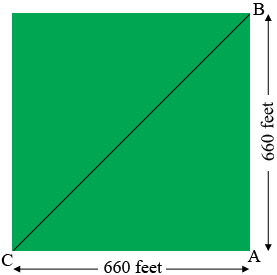SEARCH HOMEMath Central Quandaries & QueriesQuestion from Derrick, a student: I own 100 acre square of land. I want to run from farthest corner of property to farthest corner, Diagonally. How many miles would I have traveled?Hi Derrick,

One acre is 43,560 square feet so 10 acres is 435,600 square feet. If your 10 acre property is in the shape of a square then each side is of length

$\sqrt{435600} = 660 \mbox{ feet.}$Triangle $ABC$ is a right triangle. What does Pythagoras Theorem tell you about the distance from $B$ to $C?$

PennyMath Central is supported by the University of Regina and the Imperial Oil Foundation.